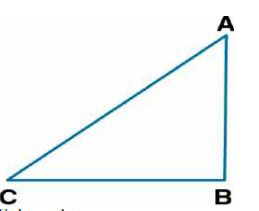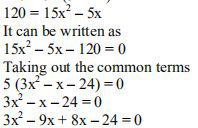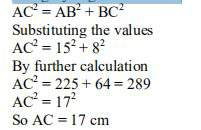# ML Aggarwal Solutions Class 9 Mathematics Solutions for Mensuration Exercise 16.1 in Chapter 16 - Mensuration

. The sides of a right-angled triangle containing the right angle are 5x cm and (3x – 1) cm. Calculate the

length of the hypotenuse of the triangle if its area is 60 \mathrm{cm}^{2}

Consider ABC as a right-angled triangle

AB = 5x cm and BC = (3x – 1) cmWe know that

Area of △ABC = ½ × AB × BC

Substituting the values

60 = ½ × 5x (3x – 1)

By further calculation

120 = 5x (3x – 1)Taking out the common terms

3x (x – 3) + 8 (x – 3) = 0

(3x + 8) (x – 3) = 0

Here

3x + 8 = 0 or x – 3 = 0

We can write it as

3x = -8 or x = 3

x = -8/3 or x = 3

x = -8/3 is not possible

So x = 3

AB = 5 × 3 = 15 cm

BC = (3 × 3 – 1) = 9 – 1 = 8 cm

In right-angled △ABC

Using Pythagoras theoremTherefore, the hypotenuse of the right angled triangle is 17 cm.

Video transcript
"hello and welcome my dear students i am rishabh the maths tutorial leader and i am here with a new question he says the sides of a right angle triangle containing the right angles are 5 x and 3 x minus 1 centimeter calculate the length of the hypotenuse of the triangle if its area is 60 centimeter square so without wasting much time let's have a look at the solution consider abc as a right angle triangle where a b is 5x and bc is 3x minus 1 centimeter we know that area of triangle abc is half a b into ac so if we substitute the values we get 60 is equals to half into 5x into 3x minus 1 so further solving it we get 120 is equals to 5x into 3x minus 1 so 120 is equals to 5 15 x square minus 5 x which further means 15 x square minus 5 x minus 120 is equals to zero now if we take out the common factor so we get 3x square minus x minus 24 is equals to zero further solving it so if you factorize it we get minus 9x plus 8x minus 24 is equals to 0 now taking out the common factors we get 3x into x minus 3 plus of 8x minus 3 is equals to 0 which further means we further get 3x plus 8 as one factor whereas the other factor has x minus 3. which is 0 so this means either 3x plus 8 is 0 or x minus 3 is 0. which further implies that x is equal to minus 8 by 3 or x is equals to 3. now since x cannot be the negative therefore the value of x equals to minus 8 is rejected and x equals to 3 is accepted so if x is equals to 3 then a b is 5 into 3 because it's 5 x which is 15 centimeter on the other hand bc is 3 into 3 minus 1 which is 8 centimeter now in right triangle abc ac square is equals to a b square plus bc square and this is using pythagoras theorem so ac is something that we need to find out which is the hypotenuse a b is 15 square plus bc is 8 square so this is 225 plus 64 this is 2 18 9 so ac is nothing but equals to 17 centimeter that means the hypotenuse of the right angle triangle is 17 centimeter so i hope my students got this question well do subscribe our channel for the regular updates you can also share your doubts in the comment section see you next time bye"
Related Questions

Lido

Courses

Teachers

Book a Demo with us

Syllabus

Maths
CBSE
Maths
ICSE
Science
CBSE

Science
ICSE
English
CBSE
English
ICSE
Coding

Terms & Policies

Selina Question Bank

Maths
Physics
Biology

Allied Question Bank

Chemistry
Connect with us on social media!